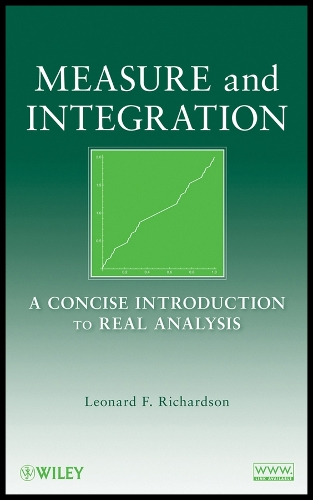•# Measure and Integration: A Concise Introduction to Real Analysis (Hardback)

(author)
£91.50
Hardback 256 Pages / Published: 21/07/2009
• We can order this

Usually dispatched within 3 weeks

A uniquely accessible book for general measure and integration, emphasizing the real line, Euclidean space, and the underlying role of translation in real analysis Measure and Integration: A Concise Introduction to Real Analysis presents the basic concepts and methods that are important for successfully reading and understanding proofs. Blending coverage of both fundamental and specialized topics, this book serves as a practical and thorough introduction to measure and integration, while also facilitating a basic understanding of real analysis. The author develops the theory of measure and integration on abstract measure spaces with an emphasis of the real line and Euclidean space. Additional topical coverage includes: Measure spaces, outer measures, and extension theorems Lebesgue measure on the line and in Euclidean space Measurable functions, Egoroff's theorem, and Lusin's theorem Convergence theorems for integrals Product measures and Fubini's theorem Differentiation theorems for functions of real variables Decomposition theorems for signed measures Absolute continuity and the Radon-Nikodym theorem Lp spaces, continuous-function spaces, and duality theorems Translation-invariant subspaces of L2 and applications The book's presentation lays the foundation for further study of functional analysis, harmonic analysis, and probability, and its treatment of real analysis highlights the fundamental role of translations. Each theorem is accompanied by opportunities to employ the concept, as numerous exercises explore applications including convolutions, Fourier transforms, and differentiation across the integral sign. Providing an efficient and readable treatment of this classical subject, Measure and Integration: A Concise Introduction to Real Analysis is a useful book for courses in real analysis at the graduate level. It is also a valuable reference for practitioners in the mathematical sciences.

Publisher: John Wiley & Sons Inc
ISBN: 9780470259542
Number of pages: 256
Weight: 472 g
Dimensions: 243 x 162 x 17 mm